## Competition may develop to unhealthy levels

When students begin to pass levels in Rocket Math, and color in the Rocket Chart in their folders, they naturally are proud of their accomplishments. Students want to tell me what level they are “on” when I visit classrooms.   Unhealthy competition may develop among students sometimes.  Some students begin to feel really bad about their slower progress, and students in the lead act arrogantly or disrespectfully.  The Rocket Math Wall Chart is designed to curb that competition.

## The Wall Chart puts all the students on the same team.Over 700 star stickers come with the Wall Chart.  Each time a student passes a level the teacher awards them with a star sticker, which they take up to the Wall Chart and put into one of the squares in the chart.  Students fill the chart from the bottom up.  The teacher sets a goal in a few weeks, which date is marked on the goal arrow, and the goal arrow is placed a couple of rows up from where the students are now.  (You can just see that in the picture above.)

## Students develop pride in their whole class.

If the students fill in the squares up to the arrow–before the date specified on the arrow–they earn a group reward such as extra recess time, or music during math, or a congratulatory note home, or a popcorn party, etc.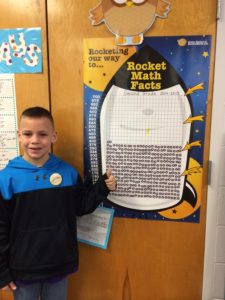In this way, each time a student passes a level they are putting up a score for the whole team.  It is good for everyone.  The teacher is able to praise the class for their hard work and accomplishments, and the whole class is able to feel good about their collective effort.

## The Wall Chart shows visitors (like principals) how well the class is doing.

Passers-by as well as interested administrators can praise the class as a whole for their successes with Rocket Math.  In many schools, classes post their completed Rocket Chart on their door with all 725 stickers in place!   The Rocket Math Wall Chart becomes a focus of pride and recognition for the whole class.  The price for the Rocket Math Wall Chart (#2005) of \$20 includes directions, plenty of star stickers, four goal arrows, and the chart itself.   They are cheaper by the dozen, \$155 for all twelve.

## The Ultimate Guide to Math Fact Fluency

Students counting on their fingers is a sure-tell sign that they didn’t acquire math fact fluency. It is sad to see students, ashamed of the only thing they know, counting on their fingers under their desks. Our elementary educational mission is failing students who haven’t developed math fact fluency, which  is the foundation to more advanced math skills.

Developing math fact fluency takes structure, organization, and work on the part of both teachers and students. In this article, I will share everything you need to know about developing math fact fluency.

## What is Math Fact Fluency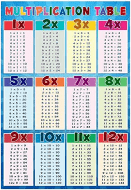Math facts are single-digit problems such as 7+9 or 6×8 or 14-5, and so on. A common name for all the multiplication math facts is the “multiplication table.” Math fact fluency is the ability to answer all math fact questions instantly from recall without having to think through the problem.

Students should be able to recall math facts instantly without having to count on their fingers or  hesitate to think about the answer. This may seem like a high bar, but our brains are great at recalling an unbelievable amount of information daily, and with practice, math facts can be recalled the same way.

## Three Reasons Why Math Fact Fluency is Important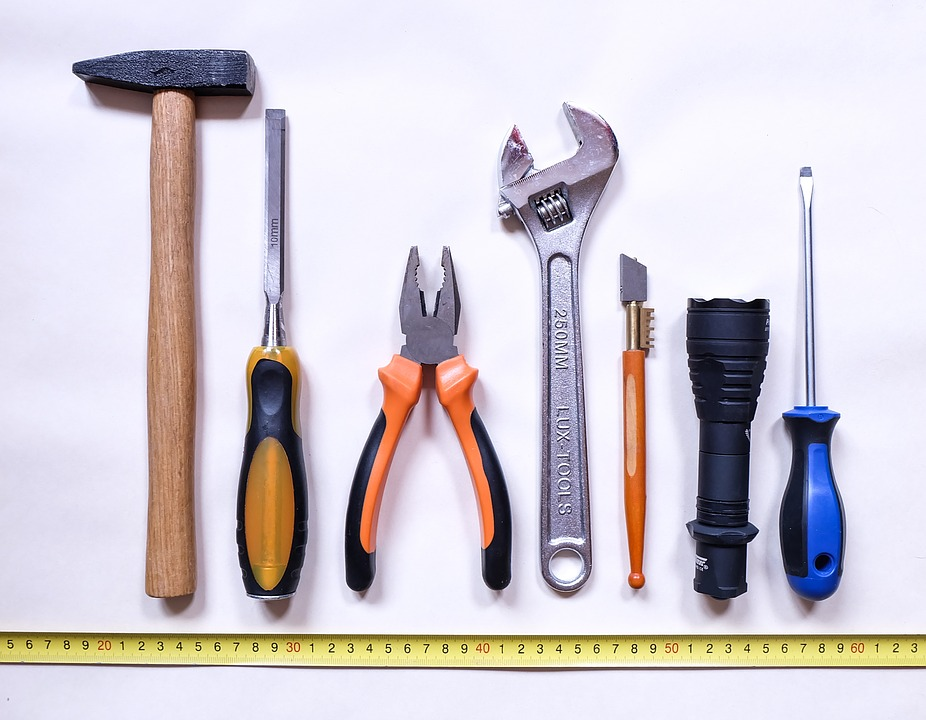Math fact fluency is critical because it is a “tool skill.” Meaning, it is a tool that is used in the process of doing other math problems. Developing this tool skill makes learning math easier as concepts get more complicated. This tool skill needs to be automatic in the student’s brain, in order to save precious short-term memory resources.

Math fact fluency can be compared to reading. Students must recognize words automatically to comprehend the author’s meaning. Otherwise, they will spend too much time decoding individual words.

When students are fluent in math facts, they are focused on the math process as a whole, rather than stopping to puzzle out the facts. This is important for three reasons:

### 1. Students with math fact fluency make fewer errors

Students who lack math fact fluency often make careless errors doing arithmetic computations . If they devote too much energy to deriving math facts, they lose sight of the problem at hand and make mistakes that would otherwise be obvious. Those who can effortlessly recall math facts can concentrate on what they are doing, and ultimately make fewer errors.

### 2. Math fact fluency makes learning math easier

When a new math procedure is introduced, students who have math fact fluency can easily follow the thread of instruction. Without this fluency, students fall behind instruction or demonstrations as they try working out math facts. This distraction takes away from a student absorbing all of the details necessary to successfully learn new math processes.

The first teacher to use Rocket Math to teach subtraction facts to her second graders realized the benefit first hand. She told me that with Rocket Math, she was able to teach regrouping in subtraction in just three days.

Her students mastered the math facts, and the outcome was extraordinary. The teacher shared that since these students had developed fluency in subtraction facts, they were able to learn other procedures easily.

### 3. Students who have developed math fact fluency enjoy math and always complete their work

Having to count on your fingers or look up facts on a timetable is slow and onerous. When students can’t work quickly, math problems become a dreaded drudgery. Students are motivated by mastering new skills, which will help them work faster and build confidence . Those who can quickly recall math facts will complete their work with ease , and enjoy the feeling of accomplishment.

## How to Build and Improve Math Fact FluencyBuilding and improving math fact fluency requires a systematic effort over the elementary years. It is a long climb to achieve mastery and there are no short-cuts.

Consistent daily practice throughout elementary school is important for retention. Slow and steady wins the race when building math fact fluency.

Math fact practice should be structured in such a way that students are learning a small number of facts at a time. These small groups of facts  should be practiced daily until students have reached mastery. As time goes on, more groups of math facts are introduced systematically in small amounts for students to master.

Learning 1s to 9s facts in the four basic operations will take elementary students months to master. Worksheets and game applications are two of the best ways to teach math fact fluency over time. Combining structured math fact learning, practice, and evaluation with fun math fact games helps students develop number sense and understand complex numerical relationships.

## Teaching Math Fact Fluency with Worksheets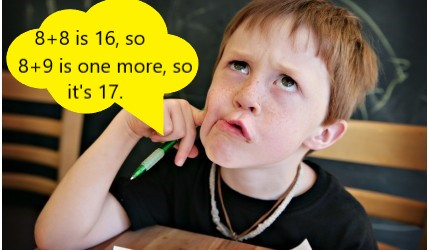Worksheets are popular tools that teachers reach for when teaching math facts, but sadly, they often fall short for the majority of students. A few select students will begin memorizing the facts on their own accord in order to make the worksheets easier, but most students will continue to slowly work out the facts either on their fingers or in their heads. These students may never develop a strong recall of the facts and become flustered when asked to answer problems on the spot.

Fortunately, there are specific worksheets that are effective in building math fact fluency. The key is having worksheets that are structured, systematic, and sequenced. Each worksheet should only have two to four facts to be learned.

By working on only two to four facts, these worksheets help teach memorization for a strong recall, rather than reinforcing working out problems slowly. Students  will then be able to remember these small groups of facts easier, and by the end of the worksheet will be writing answers from memory.

## Teaching Math Fact Fluency with The Rocket Math Worksheet Program

Rocket Math Worksheet Program improves upon this concept by using paired practice and saying facts aloud. Students partner up and practice quickly recalling facts together. One student asks the questions and watches for when their partner hesitates to answer. He or she then gives his or her partner more opportunities to practice the harder facts.

The students switch roles, and after both have answered questions, they then take a one minute test on the facts that they have learned so far. If students are answering as fast as their fingers will carry them, then they pass the level and move on to the next worksheet in the sequence.

Ten minutes of practice  every day gets the job done, especially when paired with using these facts in higher level math problems.

## Teaching Math Fact Fluency with Games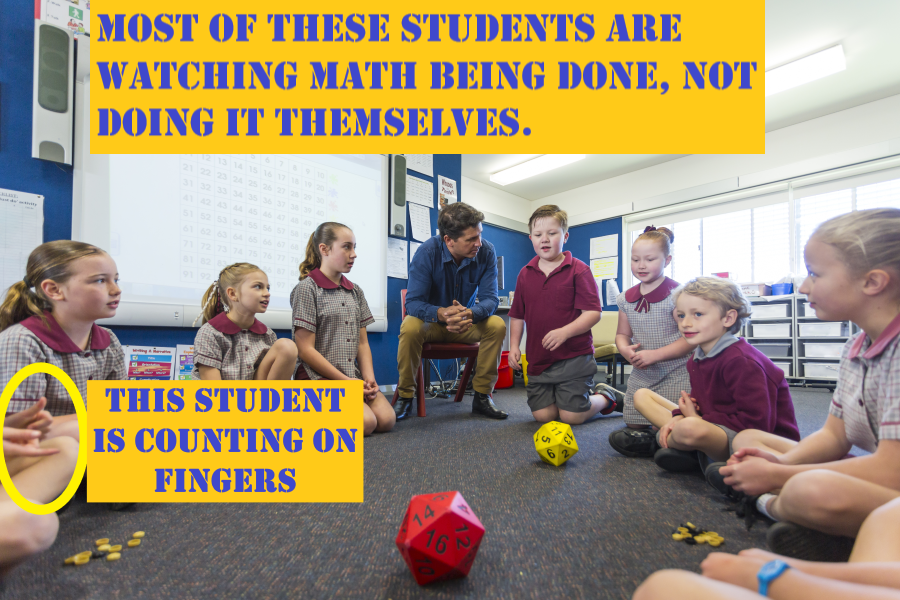In addition to worksheets, schools of education tell teachers to use games to “teach” math facts. Unfortunately, most games and fun activities do not actually help individual students learning math facts to the level of fluency. These games, such as bingo or dice, have several fallouts:

• Students spend most of their time waiting for their turn rather than practicing facts.
• They do not focus on teaching a small group of facts in a manner that helps students commit them to memory.
• The games do not adjust to an individual student’s level of fluency.
• Students can pace the game slowly enough to have time to figure out facts rather than requiring recall.
• It is difficult to keep every student engaged, as those who are behind are less likely to participate.

## Using the Rocket Math Online Game as an Effective Way to Teach Math Fact Fluency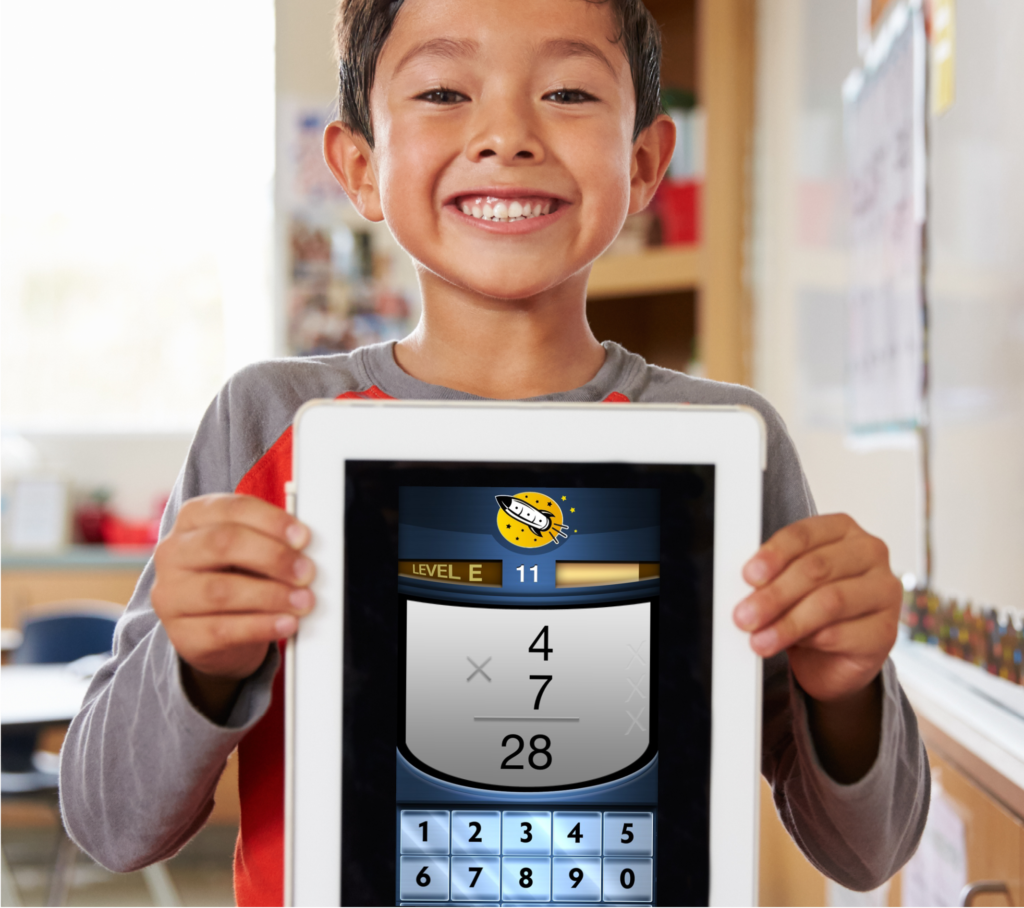There are games that are very effective at building math fact fluency. Games such as the Rocket Math Online Game have several important features that make a big difference.

1. Every student is engaged in answering math facts—not waiting for a turn.
2. Students learn only a few new facts at a time so that they can remember them.
3. The game provides lots of focused practice on each set of facts.
4. The game requires students to answer quickly, which guarantees the students recall the answer rather than “figuring it out” over and over.
5. The game gives an immediate correction and extra practice on any facts that students cannot answer quickly and correctly.
6. The game only introduces new facts once students demonstrate mastery of facts learned so far.
7. The game gives students explicit feedback so they have a sense of accomplishment as they work their way through an operation.

## Math Fact Fluency Benchmarks

The following benchmarks are reasonable expectations for a school that has an effective math fluency program in place. Of course, a student cannot write math facts any faster than they can normally write, so take that into account when looking at fluency benchmarks. Adjust the benchmarks for students who do not write quickly.

### Kindergarten Numeral Writing Fluency Benchmarks (digits)

Start of Year Mid-Year End of Year
20 digits per minute 40 digits per minute

### First Grade Numeral Writing Fluency Benchmarks (digits)

Start of Year Mid-Year End of Year
40 digits per minute 60 digits per minute 60 digits per minute

### First Grade Math Fact Fluency Benchmarks (problems per minute)

Start of Year Mid-Year End of Year

### Second Grade Math Fact Fluency (problems per minute)

Start of Year Mid-Year End of Year
Subtraction: 12 per minute Subtraction: 25 per minute

Third Grade Math Fact Fluency Benchmarks (problems per minute)
Start of Year Mid-Year End of Year
Subtraction: 30 per minute Subtraction: 30 per minute Subtraction: 30 per minute
Multiplication: 30 per minute Multiplication: 30 per minute

Fourth Grade Math Fact Fluency Benchmarks (problems per minute)
Start of Year Mid-Year End of Year
Subtraction: 35 per minute Subtraction: 35 per minute Subtraction: 35 per minute
Multiplication: 35 per minute Multiplication: 35 per minute Multiplication: 35 per minute
Division: 20 per minute Division: 35 per minute

Fifth Grade Math Fact Fluency Benchmarks (problems per minute)
Start of Year Mid-Year End of Year
Subtraction: 35 per minute Subtraction: 40 per minute Subtraction: 40 per minute
Multiplication: 35 per minute Multiplication: 40 per minute Multiplication: 40 per minute
Division: 35 per minute Division: 40 per minute Division: 40 per minute

Math Fact Fluency Assessment

Use this printable packet of free math fact fluency assessments to test your students’ skill levels relative to  the above benchmarks. This will give you a clear idea of your students’ fluency and where there is room for opportunity.

Rocket Math’s assessment packet includes a writing speed test, which helps create realistic expectations for individual students. Using the goal sheet ensures you will evaluate the individual student math fact fluency in light of their writing speed.

Rate students as:

1. Weak, needs fact work
2. Good, but fact work could help
3. Strong, fact work not needed

Special triage priority: if you have fourth-grade students and above, start with multiplication facts. Multiplication facts are essential to future success in math above fourth grade. Even if fourth graders are counting on their fingers for addition and subtraction, teach multiplication mastery first. If fourth graders move to the next grade without strong multiplication fact fluency, they will have a hard time successfully progressing through math.

## The Best Tools for Developing Math Fact Fluency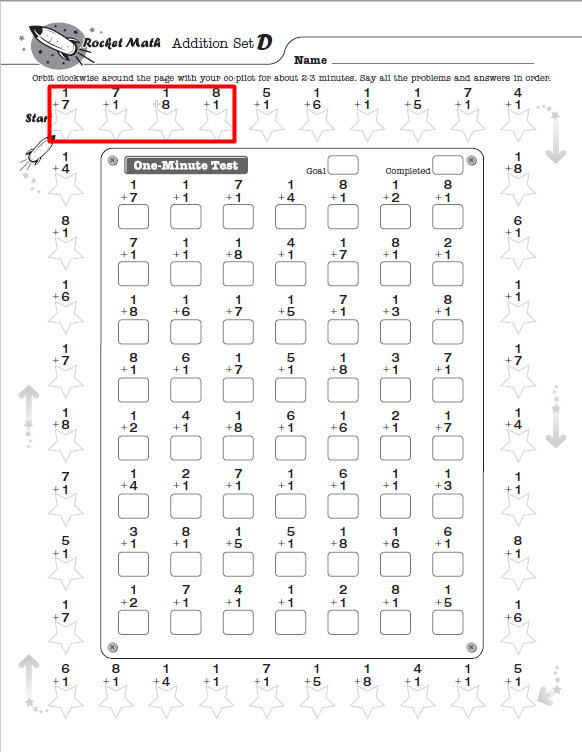With the right tools, any student can develop math fact fluency and have fun while doing it! Students use Rocket Math’s Subscription Worksheet Program to practice with partners, then take timed tests. Rocket Math also offers math facts practice online through the Rocket Math Online Game. Students can log in and play from any device, anywhere, any time of day! Start a free trial today.

Both the worksheet program and the online game help students master addition, subtraction, multiplication, and division math facts for a lifetime of success in math.

## In What Order Should Students Learn Fast Math Facts?Basic, Optional, and Alternative—there are a lot of different Rocket Math programs. But which program should you use first? And in what order should you teach fast math facts? Well, it all depends on the grade you teach and the fast math facts your students have already memorized.

## An overview of Rocket Math’s fast math fact programs

Rocket Math offers multiple programs because their are several ways to teach fast math facts. Here’s a link to a printable version of the different Rocket Math programs shown here.

### The Basic Program

Rocket Math’s basic program includes Addition, Subtraction, Multiplication, and Division (1s-9s). The basic program must be mastered by all students.

###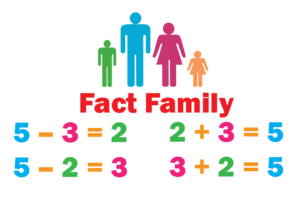The Alternative Program: Fact Families

There is another way to learn facts, which is called Fact Family math.  Instead of learning all Addition facts, students can learn Addition and Subtraction facts at the same time.  A fact family consists of four related facts, for example: 3+2 = 5, 2 + 3 = 5, 5 – 3 = 2, 5 – 2 = 3.  As an alternative to using the Basic Program, students can learn fact families up to 10 in first grade.  Then students can move on to the upper fact families 11 to 18 in second grade.  There is no clear evidence that this way is better or the separate operations way is better.  That’s why we offer both options.

### Optional Programs

The rest of the fast math facts programs like Rocket Writing for Numerals or Skip Counting are optional. You should only offer these programs to students once they have memorized the fast math facts through the Basic Program or the Alternative Program.

The only exception would be in a school where Kindergarten students did not get a chance to learn how to quickly and easily write numerals. In that case, you might take the first two months of the first grade year to run students through Rocket Writing for Numerals before beginning Addition (1s-9s).

Let’s take a closer look at how to implement each program in different grade levels.

##First grade math facts: Learn Addition

Rocket Math fast math facts programs for first graders include:

• The Basic Program
• The Alternative Program
• Fact Families (1-10) Add & Subtract
• Optional Programs
• Rocket Writing for Numerals

If first grade students are taking all year to get through sets A-Z in Addition in the Basic Program, they need some extra help.  You should intervene to help students who take more than a week to pass a level.  Often they need to practice better or practice with a better partner.  Some may need to practice a second time during the day or at home in the evening.  First grade students who finish the 1s-9s can move on to the Add to 20 Optional Program for the remainder of the year.

Likewise, if you choose to teach Fact Families (1-10) Add & Subtract from the Alternative Program instead of using the Basic Program, your students can use the Optional Programs for supplemental learning purposes.Rocket Math fast math facts programs for second graders include:

• The Basic Program
• (1s-9s) Subtraction
• The Alternative Program
• Fact Families (1-10) Add & Subtract
• Fact Families Part Two (11-18) Add & Subtract
• Optional Programs
• Subtract from 20
• Skip Counting

Second grade students must have completed Addition before starting on Subtraction (1s-9s).  They can also test out of Addition through the Placement Probes.  Second graders who cannot test out of Addition in first grade or didn’t complete it in first grade must focus on Addition.  Only after getting through Set Z of Addition should they move into Subtraction.

You can substitute the Basic Program’s (1s-9s) Addition and (1s-9s) Subtraction for the Alternative Program’s Fact Families (1-10) Add & Subtract and Fact Families Part Two (11-18) Add & Subtract.

Second grade students who complete Addition and Subtraction 1s-9s (or the Alternative Program) can move on to Subtract from 20.  Students who finish Subtract from 20 can do Skip Counting, which does a great job of preparing students to learn Multiplication facts.

## Third grade math facts: Learn Multiplication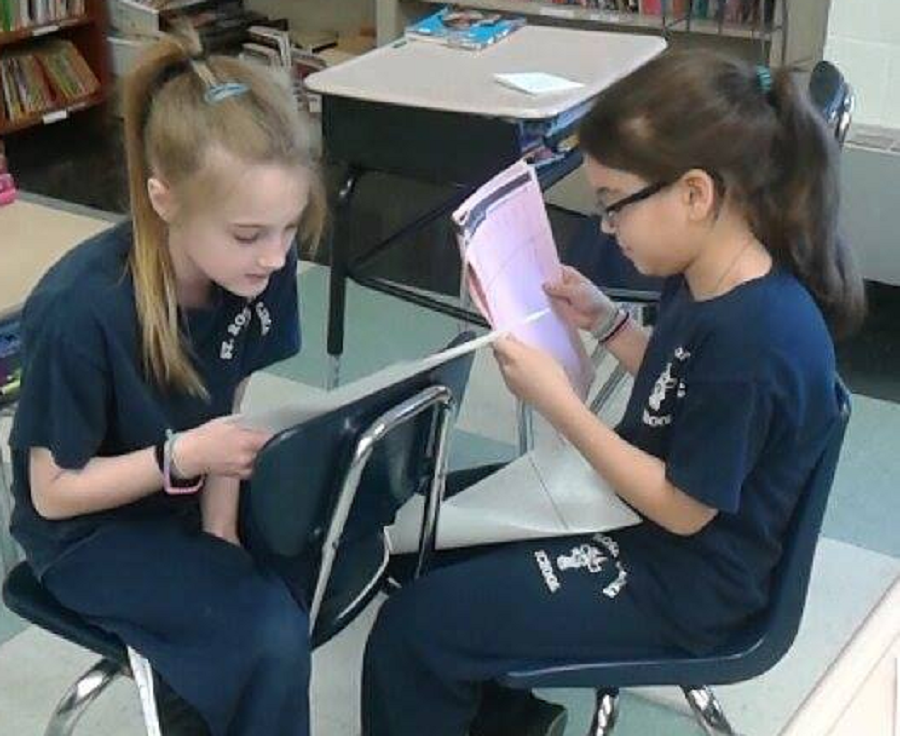There aren’t any Alternative Programs available for third graders from Rocket Math. There are only Basic and Optional Programs. These include:

• The Basic Program
• (1s-9s) Multiplication (priority)
• (1s-9s) Subtraction
• Optional Programs
• 10s, 11s, 12s Multiplication
• Factors

In third grade, Multiplication has priority—even if students have not mastered Addition and Subtraction.  Multiplication facts are so integral to the rest of higher math that students are even more crippled without Multiplication facts than they are having to count Addition and Subtraction problems on their fingers.  So do Multiplication first. Then, if there’s time, students who need to do so can go back and master Addition and Subtraction.  Once all three of these basic operations are under their belts, students can go on to 10s, 11s, 12s in Multiplication (one of the Optional Programs).  If students successfully progress through each program and there is enough time left in the school year, introduce the Factors program next.

## Fourth grade math facts: Learn Multiplication and Division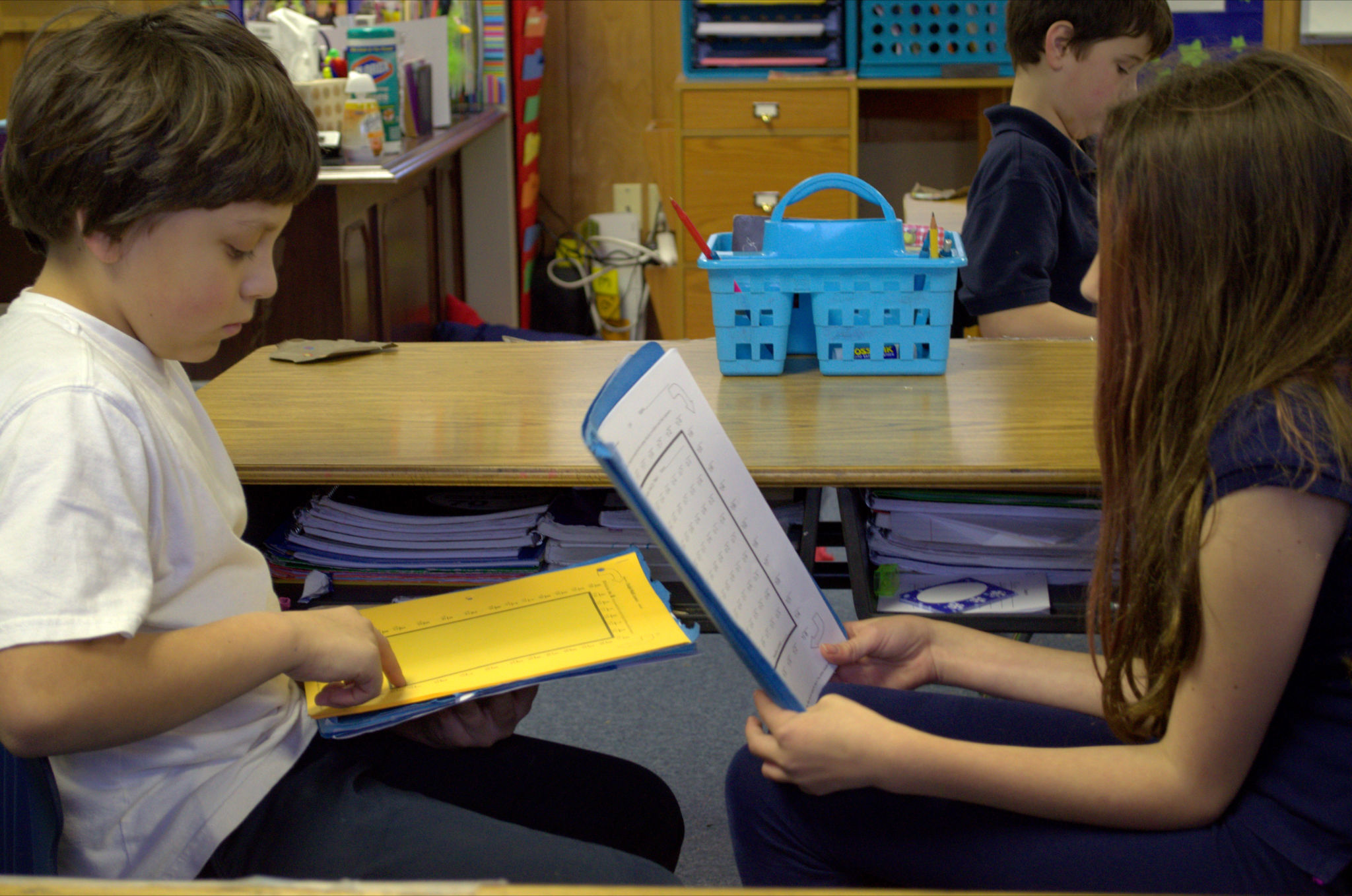Like the programs for third graders, there aren’t any Alternative Programs available for fourth graders. There are only Basic and Optional Programs, which include:

• The Basic Program
• (1s-9s) Multiplication (priority)
• (1s-9s) Division (second priority)
• Optional Programs
• 10s, 11s, 12s Multiplication
• Factors

In fourth grade, students need to have completed Multiplication before going on to Division. If they complete Division, they can go on to 10s, 11s, 12s Division, followed by Factors, and then equivalent fractions (shown in the fifth grade section below).

## Fifth grade math facts: Learn all basic operations first, then they can branch out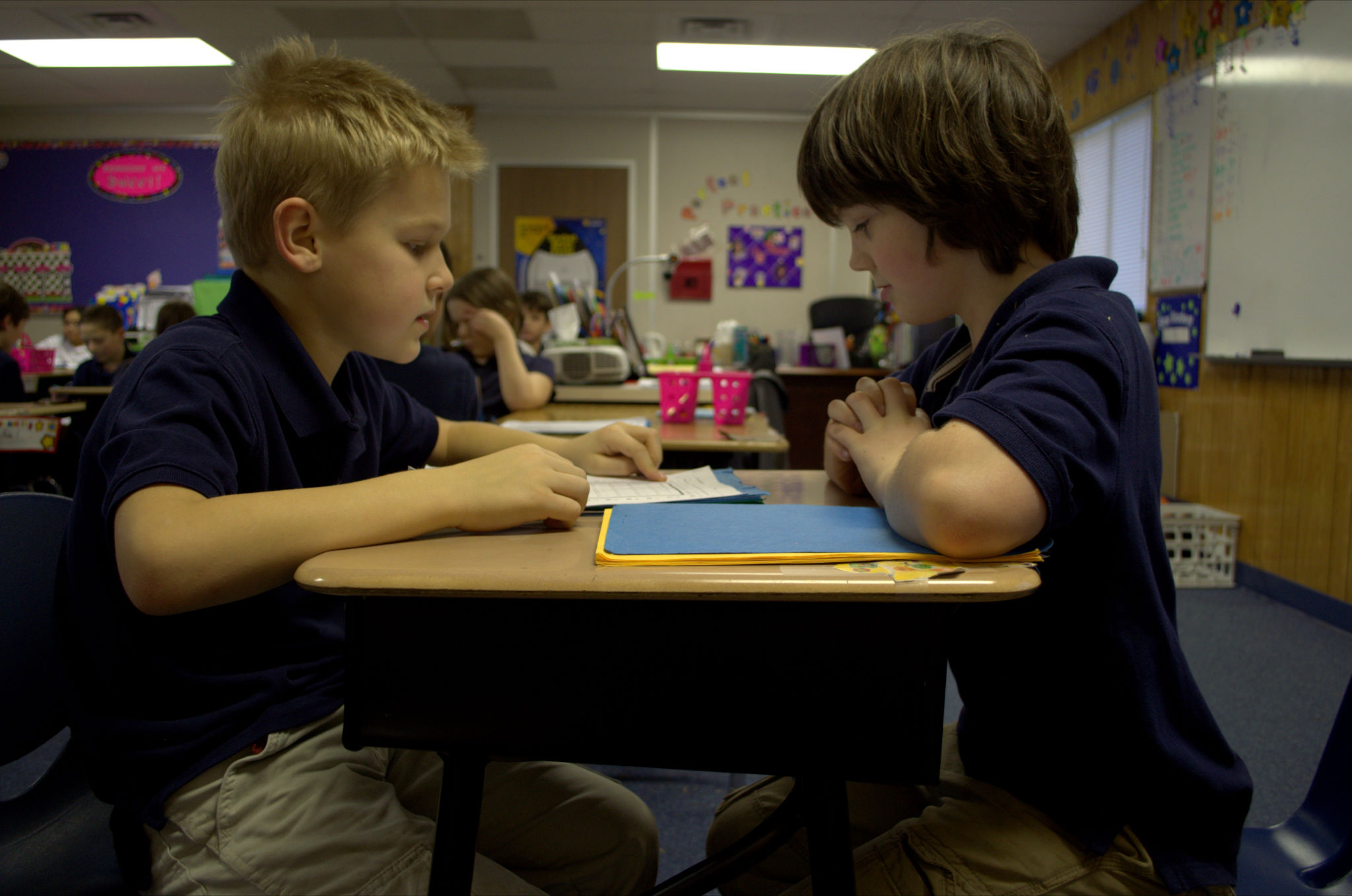By fifth grade, students should have completed all four basic operations (1s-9s) within the Basic Program (or the Alternative Program for grades one and two).  If students have not completed these basics (and cannot test out of them with the Placement Probes) then the sequence they should follow is Multiplication, followed by Division, then go back and complete Addition followed by Subtraction.  The same recommendations hold for students in any grade after fifth.

Once students have mastered the basics (1s-9s add, subtract, multiply, divide), the supplemental pre-algebra programs are recommended.  These will help more than learning the 10s, 11s, 12s facts.  I would recommend this order:

1. Factors
2. Equivalent Fractions
4. Learning to Subtract Integers or Mixed Integers

## Get a free 30-day trial of our Online Game

Here’s information (that may not be apparent) about the next step–after registering for a free account for the Rocket Math Online Game.  The next step is to to try out the game with some students by signing up for our No risk 30 day trial.

Your credit card will not be charged until the end of your 30 day trial, so if you cancel before then you do not pay a thingYou can order from the “My Profile” page of your account with a credit card to order subscriptions. It looks like this picture.

No gotcha here–See how the auto-renew is turned off by default?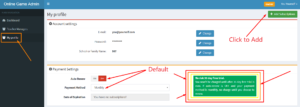Leave the renewal period set to monthly, and leave auto renew set to OFF in your profile.

### Your subscription will simply end after 30 days.

No matter how many subscriptions you order, your credit card won’t be charged until you login and renew.  So you can try the game for free to see if it’s worth paying for with no risk of being charged for it.

### Non-credit card options

If you wish to buy subscriptions by sending in a Purchase Order here’s a link to our order form. Or, if you wish to order online with either PayPal or a PO number click this link to get to that page

Either in PayPal or with a PO we will give you 13 months, and if you tell us you don’t want it during the first month, we’ll cancel your subscription and cancel the invoice. With PayPal we’ll give you a full refund if you don’t want to keep it.

If you ask, I can also manually give you a 30 day free trial–without you having to enter a payment method.  Then we can send an invoice if you wish to continue.  Just contact [email protected], with the number of subscriptions you would like to use during your free trial.

## Foolproof method for finding factors

Knowing when you’ve found ALL the factors is the hard part.

Students have to learn how to find the factors of a number because several tasks in working with fractions require students to find the factors of numbers. Thinking of some of the factors of a number is not hard. What is hard is knowing when you have thought of ALL the factors. Here is a foolproof, systematic method I recommend: starting from 1 and working your way up the numbers. This is what student practice in the Rocket Math Factors program.

Dr Don has a white board type video lesson that explains this in 6 minutes.

https://www.educreations.com/lesson/view/how-to-find-all-the-factors-of-a-number/46790401/

How to find all the factors of numbers
Always begin with 1 and the number itself-those are the first two factors. You write 1 x the number.  Then go on to 2. Write that under the 1. If the number you are finding factors for is an even number then 2 will be a factor. Think to yourself “2 times what equals the number we are factoring?” The answer will be the other factor.
However, if the number you are finding factors for is an odd number, then 2 will not be a factor and so you cross it out and go on to 3. Think to yourself “3 times what equals the number we are factoring?” There’s no easy rule for 3s like there is for 2s. But if you know the multiplication facts you will know if there is something. Then you go on to four—and so on.

The numbers on the left start at 1 and go up in value.  The numbers on the right go down in value.  You know you are done when you come to a number on the left that you already have on the right.  Let’s try an example.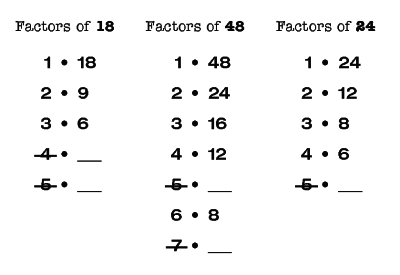Let’s find the factors of 18.  (To the left you see a part of a page from the Rocket Math factoring program.)
We start with the first two factors, 1 and 18. We know that one times any number equals itself. We write those down.
Next we go to 2. 18 is an even number, so we know that 2 is a factor. We say to ourselves, “2 times what number equals 18?” The answer is 9. Two times 9 is 18, so 2 and 9 are factors of 18.
Next we go to 3. We say to ourselves, “3 times what number equals 18?” The answer is 6. Three times 6 is 18, so 3 and 6 are factors of 18.
Next we go to 4. We say to ourselves, “4 times what number equals 18?” There isn’t a number. We know that 4 times 4 is 16 and 4 times 5 is 20, so we have skipped over 18. We cross out the 4 because it is not a factor of 18.
Next we go to 5. We might say to ourselves, “5 times what number equals 18?” But we know that 5 is not a factor of 18 because 18 does not end in 5 or 0 and only numbers that end in 5 and 0 have 5 as a factor. So we cross out the five.
We would next go to 6, but we don’t have to. If we look up here on the right side we see that 6 is already identified as a factor. So we have identified all the factors there are for 18. Any more factors that are higher we have already found. So we are done.

Now let’s do another number.  Let’s find the factors of 48.

We start with the first two factors, 1 and 48.  We know that one times any number equals itself.

Next we go to 2.  48 is an even number, so we know that 2 is a factor.  We say to ourselves, “2 times what number equals 48?”  We might have to divide 2 into 48 to find the answer is 24.  But yes 2 and 24 are factors of 48.

Next we go to 3.  We say to ourselves, “3 times what number equals 48?”   The answer is 16.  We might have to divide 3 into 48 to find the answer is 16.  But yes 3 and 16 are factors of 48.

Next we go to 4.  We say to ourselves, “4 times what number equals 48?”  If we know our 12s facts we know that 4 times 12 is 48.  So 4 and 12 are factors of 48.

Next we go to 5.  We might say to ourselves, “5 times what number equals 48?”   But we know that 5 is not a factor of 48 because 48 does not end in 5 or 0 and only numbers that end in 5 and 0 have 5 as a factor. So we cross out the five.

Next we go to 6. We say to ourselves, “6 times what number equals 48?”  If we know our multiplication facts we know that 6 times 8 is 48.  So 6 and 8 are factors of 48.

Next we go to 7.   We say to ourselves, “7 times what number equals 48?”   There isn’t a number.  We know that 7 times 6 is 42 and 7 times 7 is 49, so we have skipped over 48.  We cross out the 7 because it is not a factor of 48.

We would next go to 8, but we don’t have to.  If we look up here on the right side we see that 8 is already identified as a factor.  So we have identified all the factors there are for 48.  Any more factors that are higher we have already found.  So we are done.

## Facts practice: does it belong in middle school math?

It sure does, if you’re seeing this happen in your class!

Most middle school math teachers confide to me that their classrooms are negatively impacted by the number of students who stop to count out facts on their fingers.  Their issue was always what to do during facts practice with the other students who do know their facts.  It has taken a couple of years but I have put together a package of pre-algebra skills that are worth middle school students’ time practicing which are available in the Universal Subscription. Because the routine of Rocket Math is the same whether the students are practicing basic multiplication facts or learning equivalent fractions you’ll be able to manage all these different levels during the same ten-minute session.

Teachers know it is imperative that finger-counting middle schoolers get practice learning their facts.  Rocket Math is an excellent way to do that.  They will develop fluency and automaticity with the basic facts in an operation in a semester and from then on your lessons will be much easier.  Not only that, but a much higher proportion of the students will be finishing assignments.  There is a “Placement Probe” that can identify students who know their facts in about one minute. The students who know the basic facts of multiplication and division can be placed into the pre-algebra practice programs.

. Students probably ought to begin with the Factors program. What are the factors of 24? Answer: 1 and 24, 2 and 12, 3 and 8, 4 and 6. This is what students learn by memory from doing this program. Students practice with a partner, take a daily one minute timing, fill in a Rocket Chart, just like regular Rocket Math. Students learn all the factors for these numbers in this sequence: 12, 36, 24, 48, 18, 32, 16, 64, 10, 40, 20, 72, 8, 25, 50, 6, 21, 30, 60, 15, 45, and 100.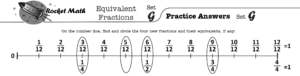EQUIVALENT FRACTIONS.  Students need to know that six-eighths is equivalent to three-fourths and that four-twelfths is equivalent to one-third.  While they can calculate these, it is very helpful to know the most common equivalent fractions by memory.  One of the most common problems students have in fractions is not “reducing their answers to simplest form.”  Equivalent fractions will help students commit 100 common equivalent fractions to memory.  Each set (A through Z) has four fractions which are displayed on a fraction number line.  Students frequently learn fractions equivalent to one,such as ten-tenths, as well as fractions that can’t be reduced, for example three-fourths is equivalent to three-fourths.  Using the fraction number line will help with student understanding of why those fractions are equivalent.

(Adding and subtracting positive and negative numbers).  Integers displays problems on a vertical number line and then teaches students two rules about how to solve problems that add or subtract positive and negative numbers.

Rule 1: Go up when you add a positive number OR subtract a negative number.
Rule 2: Go down when you subtract a positive number OR add a negative number.

Students gradually learn several variations of all four types of problems.  They practice with the number line on each page and then have a chance to build fluency on the top half of the page as they work with their partner.  You will probably not be surprised that there is a one-minute test on each set.  The goals are slightly different than before.  Students are to be 100% accurate and to complete at least 80% of their rate at answering simple addition and subtraction problems.

10s, 11s, 12s Multiplication and 10s, 11s, 12s Division facts are also available in the Universal Subscription.  If you have students who think they know the basic facts, but need review, putting them into either of these programs will review the 1s through 9s facts, teach them new ones and allow them to save face.

Among these five programs there are good things for ALL middle school math students to learn, even the more advanced students.  This will enable a math teacher to devote ten minutes a day to fact practice without holding anyone back.  Everyone will have something meaningful to practice during that time.  I think this could be a huge step forward for a lot of middle school MATH classrooms.

## Open letter to parents: Rocket Math HOME Apps

Dear Parents,

I designed the Rocket Math Apps to help children LEARN math facts such as 9 + 7 or 6 x 9.  Most apps have students randomly practice math facts, but aren’t much help in learning the facts in the first place!  The Rocket Math App is different because your child will be successful from the start and will gradually learn all the facts in each operation—without noticing!  They will play the app, but as they progress through the Levels A to Z they will LEARN all of the facts in sequence. Children will learn them well enough so that they don’t have to count on their fingers or stop to figure out the answer.  They will know the facts automatically so they can get on with the rest of learning math.  Take it from a long-time educator, they will begin to enjoy math once they stop struggling with figuring out facts, like this young man is doing.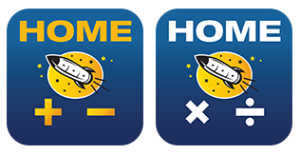Try it risk free! I don’t expect you to take my word for this.  If you download Rocket Math Addition HOME or Rocket Math Multiplication HOME you won’t have to pay up front.  Your child can try out the app and pass levels up through Level K for free.  At that point your child has already mastered at least 36 basic facts.  They are learning and probably enjoying it too!  Then if your child wants to continue playing Rocket Math, we ask that you purchase the app for \$2.99 through an in-app purchase.  See the two icons to the left.  Please look for these free versions of the Rocket Math apps with the word HOME in their name and on their icon.

Why do we have two apps?
I designed the Rocket Math Apps so that children couldn’t skip around and avoid the “hard” facts they need to learn.  The App follows the same careful sequence in the paper-and-pencil version that has worked in schools have for over 15 years.  Once a child starts on Addition, they have to follow the sequence through addition Level Z and into subtraction.  The app can keep track of up to three children as they work through the levels.  We have two apps because you may have another child who needs to work on Multiplication and Division.  Now you can download Rocket Math Multiplication HOME for that other child and your children can each proceed through their own sequence and take turns working on the same device.

Why do children have to take a 30-minute “battery recharge?”  So they continue to keep playing and learning the facts until they finish Level Z and know all the facts.  Through field testing of the Rocket Math Apps before publishing it, we found that children would keep playing for TOO LONG and wore themselves out.  (Learning is hard mental work!)  Children who played too long stopped playing the app and stopped learning!  Now that they can only play for five minutes at a time, they are happy to open the app after the break and do another five minutes of learning.   Take it from an education professor, learning that is spaced out like this, through short practice sessions over many days or weeks is THE BEST WAY TO LEARN.

Thanks!  And here’s to solving the problem of counting on your fingers to do math!

Sincerely, Dr. Don

## Do students practice sums to 12 or 18?

For addition and subtraction, do students practice with sums through 12’s or 18’s? For example, 12+ 6. Thanks.

Hi Julie!  The Rocket Math basic program (and basic subscription) is 1s through 9s both for addition and subtraction–meaning students practice single digit sums up to 9+9 and subtracting single digit numbers up to 18-9. The Rocket Math Universal subscription provides access to the Add to 20 program which includes 7 + 12 and the Subtract from 20 program which includes 19-7. The Add to 20 and Subtract from 20 programs were added because the Common Core recommends students be fluent at adding and subtracting these numbers mentally without manipulatives.

As the picture above suggests if a student knows 7+2 is 9, it doesn’t take much to learn that 7+12 is 19. However, the Add to 20 program will give students practice with those facts. Especially for students who master the 1s through 9s facts quickly, these additional programs will cement in the basic facts learning by extending them to some teen number addends. Conversely, for students who struggle with learning the 1s though 9s facts, these extra facts should be considered optional or enrichment, in my opinion.

So I recommend first graders learn the 1s-9s addition first followed by the Add to 20. Then in second grade the 1s though 9s subtraction followed by Subtract from 20. Not everyone will get through both, but kids will see the connections doing the higher problems if they have completed 1s through 9s first. They won’t need to practice to Z before they see the patterns and can do the problems without much practice. I am happy to provide this extra option for those who can take advantage of it.

## What is the Rocket Math “filing cabinet on the web?”

Can you explain the “filing cabinet on the web” a little more? Is this a place a teacher can print copies for the class or does each student have to have subscription?

A subscription gives you access to our “filing cabinet on the web.” This is a place on the web where we keep all the worksheets and a teacher goes there to print out what is needed. You just click on what you want and print it out. Each operation has its own drawer. Each drawer looks like this: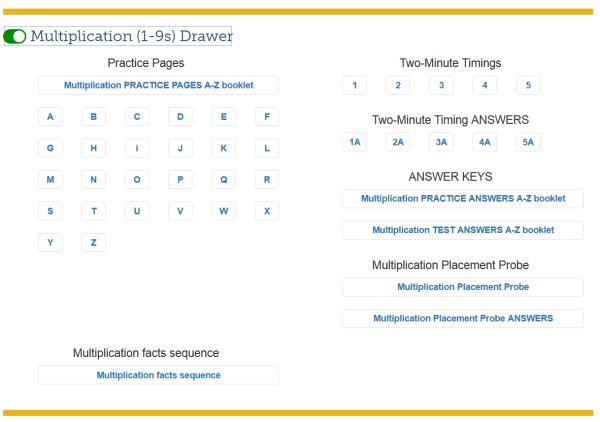There are five drawers that can be accessed with the \$29 basic subscription: Forms and Information, Addition, Subtraction, Multiplication, Division.

But wait there’s more!
There are currently 8 more drawers, the contents of which are only available to those who have the \$49 Universal subscription. The 8 programs added in the Universal Subscription are: Rocket Writing for Numerals, Skip Counting, Add to 20, Subtract from 20, Multiplication 10s, 11s, and 12s, Division 10s, 11s, and 12s, Factors, and Integers. Click on the name of any of these programs and you can get more information on that program.
You can preview (before you buy) the whole subscription site here: Rocket Math Subscription preview site.

## Challenge your students with the Race for the Stars Game Center!How can you entice your students to play the Race for the Stars game in a center?

By letting them post their record/best time for completing the game boards. Race for the Stars is a great game for students to practice their math facts. The game provides 24 problems tiles students can race to put down next to the answers in the game board as fast as they can. There is now a Game Center Kit that give you everything you need to set up a center in your room that students will want to visit. The key is the poster with room for student labels with their names. Then next to their name is a place for them to write their best time at filling the game board. Well, actually their partner with the stop watch should probably write down their time. All the students in the class can rotate through the Game Center to record how fast they can fill either or both of the two game boards. One, for levels A-K are the first facts to be learned in Rocket Math. The second game board, L-Z are the facts learned in the last half of the Rocket Math levels.

But how can you motivate your students to play the game again and again to get the practice they need?

Here is the coolest part of the Race for the Stars Game Center. Students can go back and get someone to time them filling the Race for the Stars gameboard again. If the timer with the stopwatch sees that the student beat their previous best, they get to record the new record time, AND cover the old time up with a star sticker. Getting to put up a star sticker next to your name proves you were able to beat your own previous best–that is impressive! In fact, the savvy teacher will make a daily stop to see who in the class has been able to add a star sticker to the poster next to their name, showing that they beat their previous best time.

And that is how you motivate students to use the Race for the Stars Game Center (item #2112) to practice their math facts in their spare time!